Most Affordable JEE | NEET | 8,9,10 Preparation by Kota's Top IITian Doctor Faculties

# Carbonyl Compounds - JEE Main Previous Year Questions with Solutions`
JEE Main Previous Year Papers Questions of Chemistry with Solutions are available at eSaral. Practicing JEE Mains chapter wise questions of Chemistry will help the JEE aspirants in realizing the question pattern as well as help in analyzing weak & strong areas. Simulator   Previous Years AIEEE/JEE Mains Questions
Q. Which of the following on heating with aqueous KOH, produces acetaldehyde ? (1) $\mathrm{CH}_{2} \mathrm{ClCH}_{2} \mathrm{Cl}$ (2) $\mathrm{CH}_{3} \mathrm{CHCl}_{2}$ (3) $\mathrm{CH}_{3} \mathrm{COCl}$ (4) $\mathrm{CH}_{3} \mathrm{CH}_{2} \mathrm{Cl}$ [AIEEE-2009]
Ans. (2)
Q. In Cannizzaro reaction given below :- $2 \mathrm{Ph} \mathrm{CHO}$ $\mathrm{PhCH}_{2}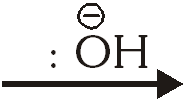\mathrm{OH}+\mathrm{PhCO}_{2}^{\Theta}$ the slowest step is :- (1) The abstraction of proton from the carboxylic group (2) The deprotonation of $\mathrm{CH}_{2} \mathrm{OH}$ (3) The attack of $: \stackrel{\ominus}{\mathrm{O}} \mathrm{H}$ at the carboxyl group (4) The transfer of hydride to the carbonyl group [AIEEE-2009]
Ans. (4)
Q. One mole of a symmetrical alkene on ozonolysis gives two moles of an aldehyde having a molecular mass of 44 u. The alkene is :- (1) Ethene (2) Propene (3) 1-Butene (4) 2-Butene [AIEEE-2010]
Ans. (4)
Q. Ozonolysis of an organic compound gives formaldehyde as one of the products. This confirms the presence of :- (1) An isopropyl group (2) An acetylenic triple bond (3) Two ethylenic double bonds (4) A vinyl group [AIEEE-2011]
Ans. (4)
Q. Ozonolysis of an organic compound 'A' produces acetone and propionaldehyde in equimolar mixture. Identify 'A' from the following compounds : - (1) 2-Methyl - 1- pentene (2) 1-Pentene (3) 2-Pentene (4) 2-Methyl-2-pentene [AIEEE-2011]
Ans. (4)
Q. Trichloroacetaldehyde was subjected to Cannizzaro's reaction by using NaOH. The mixture of the products contains sodium trichloroacetate and another compound. The other (1) 2,2,2–Trichloropropanol (2) Chloroform (3) 2,2,2–Trichloroethanol (4) Trichloromethanol [AIEEE-2011]
Ans. (3)
Q. Silver Mirror test is given by which one of the following compounds? (1) Formaldehyde (2) Benzophenone (3) Acetaldehyde (4) Acetone [AIEEE-2011]
Ans. (1,3)
Q. In the given transformation, which of the following is the most appropriate reagent ?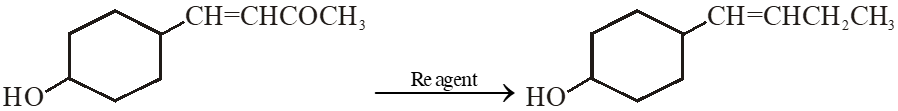(1) $\mathrm{NaBH}_{4}$ (2) $\mathrm{NH}_{2} \mathrm{NH}_{2}, \stackrel{\ominus}{\mathrm{O}} \mathrm{H}$ (3) Zn – Hg / HCl $(4) \mathrm{Na}, \mathrm{Liq.NH}_{3}$ [AIEEE-2012]
Ans. (2)
Q. Iodoform can be prepared from all except :- (1) Isobutyl alcohol (2) Ethyl methyl ketone (3) Isopropyl alcohol (4) 3-Methyl–2–butanone [AIEEE-2012]
Ans. (1)
Q. A compound with molecular mass 180 is acylated with $\mathrm{CH}_{3} \mathrm{COCl}$ to get a compound with molecular mass 390. The number of amino groups present per molecule of the former compound is :- (1) 2 (2) 5 (3) 4 (4) 6 [JEE(Main)-2013]
Ans. (2)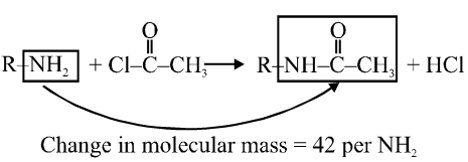180 molecular mass after acylation gives compound of molecular mass 390. Change in molecular mass = 390 – 180 = 210 so number of $\mathrm{NH}_{2}$= $\frac{210}{42}$ = 5 group
Q. The major organic compound formed by the reaction of 1, 1, 1–trichloroethane with silver powder is :- (1)2-Butyne (2) 2-Butene (3) Acetylen (4) Ethene [JEE(Main)-2014]
Ans. (1)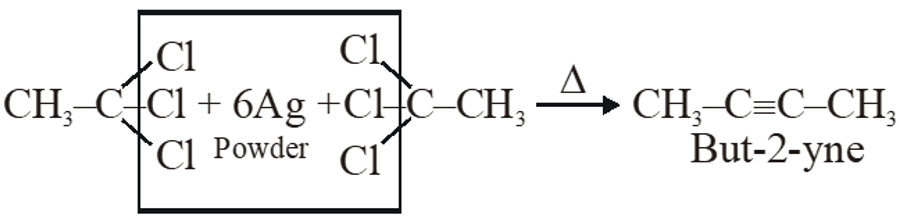Q. The most suitable reagent for the conversion of $\mathrm{R}-\mathrm{CH}_{2}-\mathrm{OH} \rightarrow \mathrm{R}-\mathrm{CHO}$ is :- (1) $\mathrm{CrO}_{3}$ (2) PCC (Pyridinium chlorochromate) (3) $\mathrm{KMNO}_{4}$ (4) $\mathrm{K}_{2} \mathrm{Cr}_{2} \mathrm{O}_{7}$ [JEE(Main)-2014]
Ans. (2)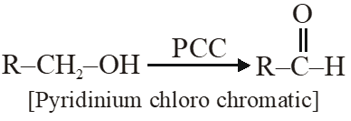PCC is milder oxidising agent, oxidises only into aldehyde.
Q. A compound A with molecular formula $\mathrm{C}_{10} \mathrm{H}_{13} \mathrm{Cl}$ gives a white precipitate on adding silver nitrate solution. A on reacting with alcoholic KOH gives compound B as the main product. B on ozonolysis gives C and D. C gives Cannizaro reaction but not aldol condensation. D gives aldol condensation but not Cannizaro reaction. A is :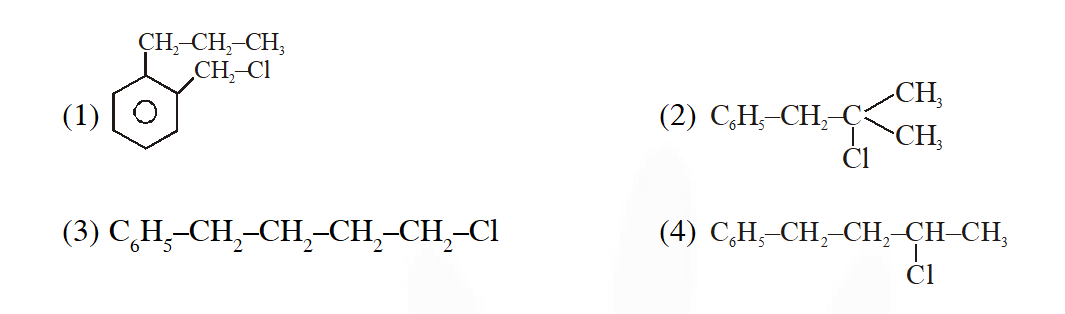#tag [JEE(Main)-2015]
Ans. (2)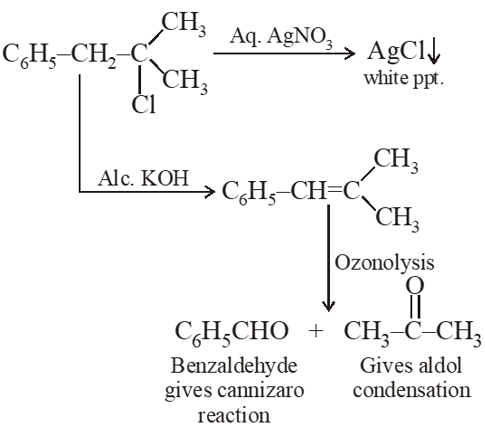Q. In the reaction sequence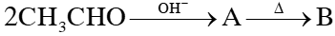; the product B is:- (1) $\mathrm{CH}_{3}-\mathrm{CH}=\mathrm{CH}-\mathrm{CHO}$ (2) $\mathrm{CH}_{3}-\mathrm{CH}_{2}-\mathrm{CH}_{2}-\mathrm{CH}_{3}$ (3) $\mathrm{CH}_{3}-\mathrm{CH}_{2}-\mathrm{CH}_{2}-\mathrm{CH}_{2}-\mathrm{OH}$ (4)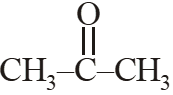[JEE(Main)-2015]
Ans. (1)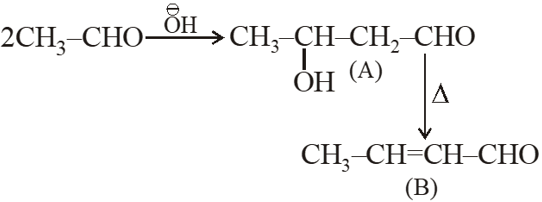Q. Which compound would give 5-keto-2-methyl hexanal upon ozonlysis?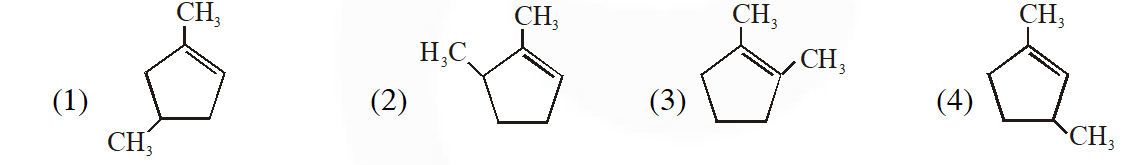[JEE(Main) 2015]
Ans. (4)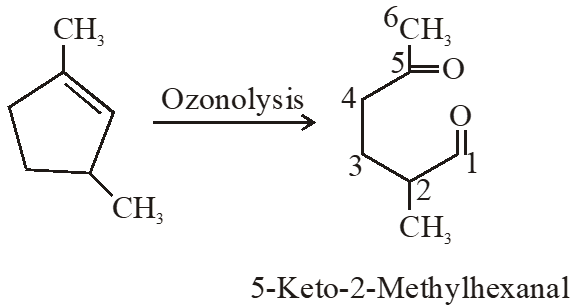Hava
Aug. 14, 2023, 6:35 a.m.
Bijlee ki raani main hu ayi,, kehete hain mujhko HAWA HAWAI.
Zakwan Shaikh
Sept. 4, 2020, 11:08 p.m.
Their services are better compared to others but we need it more coz we are able to solve it with an interest, so please I request you to add more past yr ques
Bhai
Aug. 28, 2020, 3:20 p.m.
Teri to
binod
Aug. 25, 2020, 1:20 p.m.
binod
Magar akshay anil
May 30, 2020, 1:10 p.m.
Best but we need more past year question